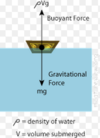# Physics 1 Flashcards Preview

## ALTIUS > Physics 1 > Flashcards

Flashcards in Physics 1 Deck (35):
1

2

3

4

5

6

7

8

9

10

11

12

13

14

15

16

17

## Inclined Planes When is the equation when solving for: Force down an IP PARALLEL to the surface?

### F=mgsinθ18

## Inclined PlanesWhen is the equation when solving for:Normal Force (FN) down an IP

### FN=mgcosθ19

## Inclined Planes When is the equation when solving for: Velocity of a particle at the base of an inclined plane What other kind of problem could this equation be used to help solve?

### Vfinal = √2ghCan also be used to help solve for FALLING OBJECT problems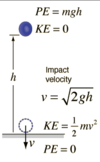20

## Inclined Planes When is the equation when solving for:  ACCELERATION DOWN an IP What other equation can you derive this from?  HINT: Notice you're solving for acceleration DOWN an IP. What other equation solves for something DOWN an IP that you know of?

### a=gsinθDerived from:  F=mgsinθ (Force DOWN an IP) F=ma, ∴ "a"=gsinθ21

## Inclined Planes Why does Vf = √2gh work for either falling bodies OR a mass on an inclined plane?  HINT:What is the above equation derived from?What is happening to an object as it goes from the point where it is dropped until hitting the ground?

### The formula Vf = √2gh is derived from CONSERVATION OF ENERGY by equating mgh to ½mv2  and solving for "v" As long as friction, air resistance, etc. are ignored (which they are), energy will be conserved in an identical way... WHETHER THE OBJECT FALLS DIRECTLY TO THE GROUND OR ROLLS DOWN A PLANE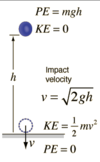22

## Inclined PlanesAs the angle of incline of a plane INCREASES: What happens to the value of a? What happens to the value of sinθ and cosθ? What happens to the normal force? What happens to the force down the plane? What is the maximum value for acceleration down an inclined plane? What is the minimum value for acceleration down an inclined plane?### 1.) Because the acceleration down a plane is directly related to the SINE of the angle(F=mgsinθ, where gsinθ​="a," since F=ma) the greater the angle, the closer the sine of the angle will be to ONE  (1.0) Because increasing the angle of SIN: 0 ⇒ 1.0 Therefore, the larger the angle, the closer the acceleration will be to 9.8m/s2 2.) The normal force is related to the cosine of the angle (Fn=mgcosθ...OSD) , so as the angle increases, this value gets closer to zero  Because increasing the angle of COS: ​ 1.0 ⇒ 0 Therefore, as the angle increases the normal force decreases 3.) The force down an inclined plane is also related to the sine of the angle so it too will increase as the angle of incline increases 4.) The theoretical maximum incline is 90 degrees ​where acceleration would be exactly 9.8 m/s2 5.) The minimum would be a plane with NO angle of incline where acceleration down the plane would be ZERO23

24

25

26

27

28

## Define "SPECIFIC GRAVITY" HINT: It's a ratio that compares two things... Give the formula for Specific Gravity

### SPECIFIC GRAVITY=A ratio that describes how DENSE something is COMPARED TO WATERSG = Dsubstance / Dwater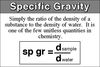29

30

31

## Define ARCHIMEDES' PRINCIPLE Differentiate b/t whether it's FULLY submerged or PARTIALLY (aka it's "floating")32

## THE BUOYANT FORCE Give the equation Describe what each part means HINT: PUG!### The Buoyant Force:Fbuoyant = ρvg v volume of fluid that GETS displaced! NOT the total volume of the fluid itself! ρ density of the fluid NOT the object!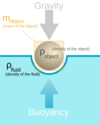33

## REMEMBER!The buoyant force is always EXACTLY EQUAL to....?

### ... to the weight of the amount of fluid that is getting DISPLACED BY the objectSo, if an object is displacing 4 lb worth of fluid, then the buoyant force would be 4 N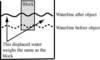34

## The Buoyant Force What causes the buoyant force?

### The best way to intuit buoyant force is to look at the pressure differential between the top and bottom of an object The fluid pressure, ρgh, will be larger at the object’s bottom surface than it is at the top surface due to the larger value of h (since it's at a deeper surface) Let’s examine a submerged cube with the same surface area both top and bottomThe formula P = F/A tells us: If pressure is greater at the bottom, and area stays the same: ​there must be a greater force UP on the bottom surface than there is down on the top surface This makes it logical that any submerged object will experience a net upward force-- because of the PRESSURE DIFFERENTIAL!!35

## The Buoyant Force How does the buoyant force change with depth (h) ? How does the buoyant force change with the mass (m) of the object? HINT: Think of the formula..."FB PUG" (facebook pug)

### 1.) Buoyant force does NOT change with depth! Depth (h) is NOT in the formula ( F = ρvg ) Therefore, we can confidently conclude that depth does NOT change buoyant force Whether the depth is shallow or very large, the pressure difference between ρgh-top and ρgh-bottom will remain the SAME 2.) Buoyant force does NOT change with depth! Similarly, we can say that the mass of the object does NOT affect buoyant force Like depth, it is NOT in the formula Nor is it accounted for by our understanding of what causes the buoyant force Remember: It's all about u